Next: Lorentz Transformation Up: Relativity and Electromagnetism Previous: Introduction

# Relativity Principle

Physical phenomena are conventionally described relative to some frame of reference that allows us to define fundamental quantities such as position and time. Of course, there are very many different ways of choosing a reference frame, but it is generally convenient to restrict our choice to the set of rigid inertial frames. A classical rigid reference frame is the imagined extension of a rigid body. For instance, the Earth determines a rigid frame throughout all space, consisting of all those points that remain rigidly at rest relative to the Earth, and to one another. We can associate an orthogonal Cartesian coordinate system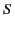with such a frame by choosing three mutually orthogonal planes within it, and measuring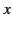,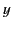, and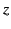as perpendicular distances from these planes. A time coordinate must also be defined, in order that the system can be used to specify events. A rigid frame, endowed with such properties, is called a Cartesian frame. The description given previously presupposes that the underlying geometry of space is Euclidian, which is reasonable provided that gravitational effects are negligible (as we shall assume to be the case). An inertial frame is a Cartesian frame in which free particles move without acceleration, in accordance with Newton's first law of motion. There are an infinite number of different inertial frames, moving with some constant velocity with respect to one another.

The key to understanding special relativity is Einstein's Relativity Principle, which states that:

All inertial frames are totally equivalent for the performance of all physical experiments.
In other words, it is impossible to perform a physical experiment that differentiates in any fundamental sense between different inertial frames. By definition, Newton's laws of motion take the same form in all inertial frames. Einstein generalized this result in his special theory of relativity by asserting that all laws of physics take the same form in all inertial frames.

Consider a wave-like disturbance. In general, such a disturbance propagates at a fixed speed with respect to the medium in which the disturbance takes place. For instance, sound waves (at STP) propagate at 343 meters per second with respect to air. So, in the inertial frame in which air is stationary, sound waves appear to propagate at 343 meters per second. Sound waves appear to propagate at a different speed in any inertial frame that is moving with respect to the air. However, this does not violate the relativity principle, because if the air were stationary in the second frame then sound waves would appear to propagate at 343 meters per second in that frame as well. In other words, exactly the same experiment (e.g., the determination of the speed of sound relative to stationary air) performed in two different inertial frames of reference yields exactly the same result, in accordance with the relativity principle.

Consider, now, a wave-like disturbance that is self-regenerating, and does not require a medium through which to propagate. The most well-known example of such a disturbance is a light wave. Another example is a gravity wave. According to electromagnetic theory, the speed of propagation of a light wave through a vacuum ismeters per second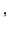(1618)

where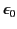and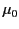are physical constants that can be evaluated by performing two simple experiments which involve measuring the forces of attraction between two fixed changes and two fixed parallel current carrying wires. According to the relativity principle, these experiments must yield the same values forandin all inertial frames. Thus, the speed of light must be the same in all inertial frames. In fact, any disturbance that does not require a medium to propagate through must appear to travel at the same speed in all inertial frames, otherwise we could differentiate inertial frames using the apparent propagation speed of the disturbance, which would violate the relativity principle.Next: Lorentz Transformation Up: Relativity and Electromagnetism Previous: Introduction
Richard Fitzpatrick 2014-06-27